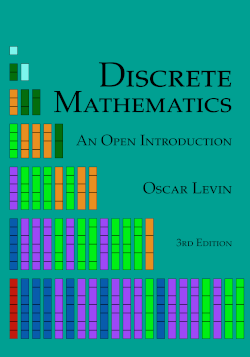# Discrete Mathematics: An Open Introduction, 3rd edition

## Chapter2Sequences

###### Investigate!
There is a monastery in Hanoi, as the legend goes, with a great hall containing three tall pillars. Resting on the first pillar are 64 giant disks (or washers), all different sizes, stacked from largest to smallest. The monks are charged with the following task: they must move the entire stack of disks to the third pillar. However, due to the size of the disks, the monks cannot move more than one at a time. Each disk must be placed on one of the pillars before the next disk is moved. And because the disks are so heavy and fragile, the monks may never place a larger disk on top of a smaller disk. When the monks finally complete their task, the world shall come to an end. Your task: figure out how long before we need to start worrying about the end of the world.
1. First, let’s find the minimum number of moves required for a smaller number of disks. Collect some data. Make a table.
2. Conjecture a formula for the minimum number of moves required to move $$n$$ disks. Test your conjecture. How do you know your formula is correct?
3. If the monks were able to move one disk every second without ever stopping, how long before the world ends?
This puzzle is called the Tower of Hanoi. You are tasked with finding the minimum number of moves to complete the puzzle. This certainly sounds like a counting problem. Perhaps you have an answer? If not, what else could we try?
The answer depends on the number of disks you need to move. In fact, we could answer the puzzle first for 1 disk, then 2, then 3 and so on. If we list out all of the answers for each number of disks, we will get a sequence of numbers. The $$n$$th term in the sequence is the answer to the question, “what is the smallest number of moves required to complete the Tower of Hanoi puzzle with $$n$$ disks?” You might wonder why we would create such a sequence instead of just answering the question. By looking at how the sequence of numbers grows, we gain insight into the problem. It is easy to count the number of moves required for a small number of disks. We can then look for a pattern among the first few terms of the sequence. Hopefully this will suggest a method for finding the $$n$$th term of the sequence, which is the answer to our question. Of course we will also need to verify that our suspected pattern is correct, and that this correct pattern really does give us the $$n$$th term we think it does, but it is impossible to prove that your formula is correct without having a formula to start with.
Sequences are also interesting mathematical objects to study in their own right. Let’s see why.